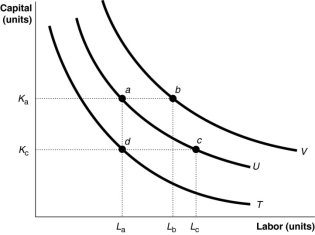• FAQ
• Contact/ Homework Answers / Economics / 11.7   Appendix: Using Isoquants and Isocost Lines to Understand Production
Not my Question
Flag Content

# Question

11.7   Appendix: Using Isoquants and Isocost Lines to Understand Production and Cost

1) An isoquant shows

A) the combinations of two goods that yield the same total satisfaction.

B) the combinations of two inputs that yield the same total product.

C) the combinations of two inputs that cost the same total quantity of money.

D) the combinations of two goods that cost the same amount of money.

2) The slope of an isoquant measures

A) the price ratio of the two inputs.

B) the average product of labor.

C) the ratio of the marginal utility of the two inputs.

D) the rate at which inputs can be substituted for each other keeping total output constant.

3) The marginal rate of technical substitution is

A) the rate at which a firm is able to substitute one input for another, while keeping total cost constant.

B) the rate at which a firm is able to substitute one input for another, while keeping the level of output constant.

C) the rate at which a firm is able to institute positive technological changes to its production process.

D) the rate at which a firm is able to increase its output by replacing labor with technology.

4) An isocost line shows

A) all the possible combinations of two inputs that a firm can use to produce its output.

B) all the possible combinations of two inputs a firm can use that have the same total cost.

C) all the possible combinations of two inputs a firm can use that have the same marginal cost.

D) all the possible combinations of two inputs with constant returns to scale.

5) The absolute value of the slope of an isocost line equals the ratio of

A) the marginal productivities of the two inputs.

B) the prices of the two inputs.

C) the marginal utilities of the two inputs.

D) the quantities of the two inputs.

6) Higher isocost lines correspond to higher

A) profits.

B) total costs of production.

C) input prices.

D) sales revenue.

7) You own a business that answers telephone calls for physicians after their offices close. You have an incentive to substitute capital for labor if the

A) price of capital increases.

B) price of labor decreases.

C) price of labor increases.

D) marginal product of labor increases.

8) Suppose the price of capital and labor remain constant but that the average educational level of workers has increased and therefore, productivity of labor increases. This would lead a firm

A) to adopt a more capital-intensive production technology.

B) to adopt a more labor-intensive technology.

C) to keep its output and production technology unchanged, but to use fewer units of labor.

D) to use only labor to produce the product.

Figure 11-129) Refer to Figure 11-12.  The movement from isoquant T to isoquant U depicts

A) a change in preferences with regards to input usage.

B) an increase in the cost of production.

C) an increase in output.

D) an increase in labor usage holding capital and output constant.

10) Refer to Figure 11-12.  Which of the following statements about the input combinations shown in the diagram is false?

A) The firm produces a higher output level when it uses input combination b compared to input combination a.

B) The firm produces a lower output level when it uses input combination d compared to input combination a.

C) The firm produces the same output level when it uses input combination a or c.

D) The firm incurs the same total cost when it uses input combination a or c to produce a given quantity of output.

## Solution 5 (1 Ratings )

Solved
Economics 2 Months Ago 46 Views